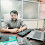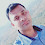# Multidimensional DICTIONARY?

49

Using this we can create dictionary keys to contain list, tuple, set, and dictionary objects to represent multiple data. It is used to manage the record of multiple entities using single objects.
Syntax of Multidimensional Dictionary:-
var = {'key': list | set | dict,...}
s = {'A':[101,102,103],'B':[34,56,78]}
Now I will contain multiple employee records and display them.
Solution:-
emp = {"empid":[1001,1002,1003],"empname":["xyz","abc","mno"],"job":["mgr","cleark"]}
for key in emp:
print(key)
for val in emp[key]:
print(val,end=' ')
print()
Dictionary can contain List, Tuple, Set, and Dictionary type Objects?
1)  Dictionary to List
emp = {"empid":[1001,1002,1003],"empname":["xyz","abc","mno"],"job":["mgr","cleark"]}
Display elements:-
for key in emp:
print(key)
for val in emp[key]:
print(val,end=' ')
print()
2)  Dictionary to Tuple
emp = {"empid":(1001,1002,1003),"empname":("xyz","abc","mno"),"job":("mgr","cleark")}
Display elements:-
for key in emp:
print(key)
for val in emp[key]:
print(val,end=' ')
print()
3)  Dictionary to Set
emp = {"empid":{1001,1002,1003},"empname":{"xyz","abc","mno"},"job":{"mgr","cleark"}}
Display elements:-
for key in emp:
print(key)
for val in emp[key]:
print(val,end=' ')
print()
4)  Dictionary to Dictionary
emp = {"branch1":{"empid":1001,"empname":"Jay Kumar"},"branch2":{"empid":1002,"empname":"Manish Kumar"}}
Display elements:-
for key in emp:
print(key)
for val in emp[key]:
print(val,emp[key][val],end=' ')
print()
Another Example of Dictionary to Dictionary and List:
student = {"A":{"rno":[1001,1003,1005],"sname":["Manish","Jay","Ravi"]},"B":{"rno":[2001,2003,2005],"sname":["Sachin","Vijay","Kavi"]}}
print(student["A"]["rno"])
for s in student:
print(s,end=' ')
for d in student[s]:
for e in student[s][d]:
print(e,end=' ')
print()
5)  Dictionary to all:-
we can store any type of objects under a dictionary key
emp = {"branch1":{"empid":1001,"empname":"Jay Kumar"},"branch2":[1002,"Manish Kumar"]}
for key in emp:
print(key)
for val in emp[key]:
if(type(emp[key]).__name__=='dict'):
print(val,emp[key][val],end=' ')
else:
print(val,end='')
print()

## ASSIGNMENT OF DICTIONARY?

create a multi-dimension dictionary to store five student records and display max, min, total fees of students.
rno name branch fees
1001 xyz  CS       45000
1002 abc   IT       22000
The solution of This Program:-
stu = {'rno':[1001,1002],'sname':['manish','ramesh'],'branch':['CS','IT'],'fees':[45000,12000]}
for key in stu:
print(key,end=' ')
print()
for j in range(0,len(stu['rno'])):
print(str(stu['rno'][j])+" "+stu['sname'][j]+" "+stu['branch'][j]+" "+str(stu['fees'][j]))
Assignment of Dictionary Objects:-
WAP to convert a dictionary to set and set to the dictionary.
WAP to convert a dictionary to list and list to the dictionary.
WAP to create a multi-dimensions dictionary?
WAP to create a multi-dimension set?
Solution:-
stu = set({2,3,4,5,6,7})  # set to set, set to list, set to tuple, set to dict
for k in stu:
print(k)
WAP to convert Multi dimension Dictionary to Set?
The solution to this assignment:-
stu = {"a":{"a1":10,"a2":20},"b":{"b1":100,"b2":200}}
set1 = set()
set2 = set()
set3 = set()
for k in stu:
print(k)
for j in stu[k]:
print(j,stu[k][j])
print(set1, set2, set3)
WAP to convert set to a dictionary?  means create a dictionary under a set
WAP to convert set to a tuple?
WAP to convert tuple to set?
WAP to convert set to list?
WAP to create Mark-sheet Program using Dictionary?
Tags

1.#convert dicitonary to list
dict={'one':1,'two':2,'three':3,'four':4}
list1=[]
list2=[]
for i in dict:
list1.append(i)
list2.append(dict[i])
print(list1,list2)

2.#convert dicitonary to set
dc={'one':1,'two':2,'three':3,'four':4}
s=set()
s1=set()
for i in dc:
print(s,s1)

3.#set to dictionary
s={1,2,3,4,5,6,7}
d={k:'hi' for k in s}
print(d)

4.#list to dicitonary
l=['one',12,'two',14,'three',14]
d={k:'a' for k in l}
print(d)

1.create two list one for key and another one for value

5.#WAP to create a multi-dimensions dictionary?
data={'no':[1,2],'name':['a','b'],'post':[3,4],'salary':[20,40]}
for i in data:
print(i,end=' ')
print()
for j in range(0,len('no')):
print(str(data['no'][j])+' '+str(data['name'][j])+' '+str(data['post'][j])+' '+str(data['salary'][j]))
print()

6.#WAP to create a multi-dimension set?
a = { {2, 4, 6, 8 }, {2, 3, 5, 7 }}

for i in range(len(a)) :
for j in range(len(a[i])) :
print(a[i][j], end=" ")
print()
doubt-error occur when run this program
a = { {2, 4, 6, 8 }, {2, 3, 5, 7 }}
TypeError: unhashable type: 'set'
>>>

7.#wap to convert set to a tuple
s={'a',1,2,'b',40}

print("before conversion",s)
tup=tuple(s)

print("after conversion",tup)

8.#tuple to set
tup=(1,2,3,'hi','hello')
a=type(tup)
print("before conversion",a,tup)
s=set(tup)
b=type(s)
print("after conversion",b,s)

9.#wap convert set to list
s={'a',1,2,'c'}
l=[]
for i in s:
l.append(i)
print(l)

10."""Q) WAP to Calculate Marksheet using five different subjects with the following condition.
1) all subject marks should be 0 to 100.
3) if all subject marks are >=33 then percentage and division should be calculated.
4) if a student is suppl then five bonus marks can be applied to be pass and the result will be "pass by grace".
5) Display Grace Subject name, distinction subject name,suppl subject name, and failed subject name.
""""

dict={'phy':90,'che':40,'emg':89,'maths':29,'hindi':89}
count=0
gm=0
s=''
dist=''
for i in dict:
if dict[i]>=0 and dict[i]<=100:
print(dict[i])
if dict[i]<33:
count=count+1
s=s+i+ " "
gm=dict[i]
else:
if dict[i]>=75:
dist = dist+ i + " "
else:
print("All subject marks should be 0 to 100")
break

total=total+marks[i]
else:
if count==0 or(count==1 and gm>=29):
per= total/5
if per>33 and per<45:
print("Third division with {0} %".format(per))
elif per<60:
print("Second division with {0} %".format(per))
else:
print("First division with {0} %".format(per))

if gm>0:
print("You are pass by grace and grace marks is ",str(33-gm), "grace subject is ",s)
if dist!=0:
print("Distinction subject name is ",dist)

elif count==1:
print("suppl in ",s)
else:
print("fail in ",s)

#sir this program not run properly

11.#WAP to convert Multi dimension Dictionary to Set?
s=set()
s1=set()
stu = {'rno':[1001,1002],'sname':['manish','ramesh'],'branch':['CS','IT'],'fees':[45000,12000]}

for key in stu:
print(s)
for s1 in range(0,len(stu['rno'])):
print(str(stu['rno'][s1])+" "+stu['sname'][s1]+" "+stu['branch'][s1]+" "+str(stu['fees'][s1]))

#output of this program is
{'rno', 'fees', 'branch', 'sname'}
1001 manish CS 45000
1002 ramesh IT 12000
>>>not appropiate output come

12.Program to convert set to dictionary-->>

s={"c","c++","vb",".net","php","python","java","testing"}
dic={y:"scs100" for y in s}
print(dic)

13.Program to convert dictionary to list-->>

dic={'one':100,'two':99,'three':98,'four':97}
l1=[]
l2=[]
for i in dic:
l1.append(i)
l2.append(dic[i])
print(l1,l2)

14.Program to convert list to dictionary-->>

l1=["a","b","c","d"]
l2=[324,361,400,441]
l=l1+l2
dic={k:"squares" for k in l}
print(dic)

DOUBT-->>Sir output should be like this-->>
{'a': 'squares', 'b': 'squares', 'c': 'squares', 'd': 'squares', 324: 'squares', 361: 'squares', 400: 'squares', 441: 'squares'}

15.Program to create multi dimension list-->>

stu={'rollno.':[11,15],'sname':['suresh','ramesh'],'branch':['ec','mech'],'fees':[65000,66000]}

for key in stu:
print(key,end=' ')
print()
for j in range(0,len(stu['rollno.'])):
print(str(stu['rollno.'][j])+" "+stu['sname'][j]+" "+stu['branch'][j]+" "+str(stu['fees'][j]))

16.Program to create multi dimension set-->>

stu=set((2,4,6,8,4,9))#set to set
for k in stu:
print(k)

Similarly
stu=set({2,4,6,8,4,9})#set to dictionary
stu=set([2,4,6,8,4,9])#set to list
stu=set((2,4,6,8,4,9))#set to tuple

17.Program to convert multi dimension dict to set-->>

stu={"a":{"a1":101,"a2":201},"b":{"b1":999,"b2":998}}
set1=set()
set2=set()
set3=set()
for k in stu:
print(k)
for j in stu[k]:
print(j,stu[k][j])

print(set1,set2,set3)

output-
a
a1 101
a2 201
b
b1 999
b2 998
{'a', 'b'} {'b1', 'b2', 'a1', 'a2'} {201, 101, 998, 999}

18.Program to convert set to tuple-->>

s={2,54,"php","python","java","testing"}

t=tuple(s)
print(t)

output->('testing', 2, 54, 'java', 'php', 'python')

19.Program to convert tuple to set-->>

t=("c","c++",111,222,78.90)
s=set(t)

print(s)

output->
{'c++', 78.9, 111, 'c', 222}

20.program to convert set to list-->>

s={"dhoni","rohit","shubham",99}
l=list(s)
print(l)

21.# Multi dimension dictionary
x = {"name":["Sunil","Anil"],"rno":[101,102], "branch":["cs","it"]}

for i in x:
print(i, end = ' ')
print()

for j in range(0,len(x["name"])):
print(str(x["name"][j]) + " " +str(x["rno"][j])+ " "+ str(x["branch"][j]))

22.#WAP to convert a dictionary to set and set to the dictionary.

a = set()
b= set()

for i in x:
print(a)

for j in range(0,len(x["name"])):
print(b)

23.#WAP to convert set to a tuple?
a = {245,58,69,64}
print(a,type(a))
y=tuple(a)
print(y,type(y))

24.#WAP to convert tuple to set?
a = (23,"abc",65,89)
print(a,type(a))
z= set(a)
print(z,type(z))
#######################

b=set()
for i in a:
print(b,type(b))

25.#WAP to convert set to list?
a = {23,45,67,89}
print(a,type(a))
b= list(a)
print(b,type(b))

################################

c=[]
for i in a:
c.append(i)
print(c,type(c))

26.# Program for Multi-Dimension Dictionary to Store Student Records and Display Max, Min, Total Fees of Students.

Student = dict()
ids = int(input("How many Student Entries you want to add:-\t"))

for j in range(0, ids):
i = input("Enter the Roll No. of Student :-")

Student[i] = {}
Student[i]['Name'] = str(input("Enter the name of Student :-")).upper()
Student[i][' Branch'] = str(input("Enter the Branch of Student:-")).upper()
Student[i]['Fee'] = int(input("Enter the Fee of Student :-"))

lst = Student.items()

res = sorted(Student.items(), key = lambda x: x['Fee'])
print(res)

i = input("Enter the Roll No. of Student :-")

if i in Student:
print("\n",Student[i])
else:
print("Wrong Roll No.")

27.# wap to dictionary to set
stu={}
set1=set()
set2=set()
dic=int(input("Enter the number or item in dictionary "))
for j in range(0,dic):
key=input("Enter the key ")
value=input("Enter the value ")
stu.update({key:value})
for i in stu:

print(set1, set2)

28.# WaP To dictionary to list
stu={}
set1=[]
set2=[]
dic=int(input("Enter the number or item in dictionary "))
for j in range(0,dic):
key=input("Enter the key ")
value=input("Enter the value ")
stu.update({key:value})
for i in stu:
set1.append(i)
set2.append(stu[i])

print(set1, set2)

29.WAP to convert a dictionary to set and set to the dictionary.

d = {"A":12,"C":34,"B":11,"E":78,"F":22}
set1=set()
set2=set()
for i in d:
print(set1)
print(set2)

set1={'F', 'A', 'B', 'C', 'E'}
d={}
for x in set1:
d.update({x:"hi"})
print(d)
dict1={x:"hi" for x in set1}
print(dict1)

30.WAP to convert a dictionary to list and list to the dictionary.

d = {"A":12,"C":34,"B":11,"E":78,"F":22}
l1=[]
l2=[]
for i in d:
l1.append(i)
l2.append(d[i])
print(l1)
print(l2)

a=['A', 'C', 'B', 'E', 'F']
d={}
for i in a:
d.update({i:"hi"})
print(d)

31.WAP to create a multi-dimensions dictionary

x=int(input("enter number of records"))
srn=[]
name=[]
fees=[]
for i in range(0,x):
s=int(input("enter srn"))
srn.append(s)
t=(input("enter name"))
name.append(t)
u=int(input("enter fees"))
fees.append(u)
dic={"srn":srn,"name":name,"fees":fees}
print(dic)

32.WAP to create a multi-dimension set

a=(1,2,3)
b=(4,5,6)
c=(7,8,9)
s={a,b,c}
print(s)

33.WAP to convert set to a tuple

set1={'F', 'A', 'B', 'C', 'E'}
t=tuple(set1)
print(t)

34.WAP to convert tuple to set

t=(1,2,3,4,5)
s=set(t)
print(s)

35.WAP to convert set to list

set1={'F', 'A', 'B', 'C', 'E'}
l=list(set1)
print(l)

36.WAP to create Mark-sheet Program using Dictionary

x=int(input("enter number of students"))
dic={}
for i in range(0,x):
n=input("enter name")
e=int(input("enter marks in english"))
m=int(input("enter marks in math"))
dic.update({n:({"english":e,"math":m})})
print(dic)
for i in dic:
print(i)
for j in dic[i]:
print (j,end=" ")
print (dic[i][j])

37.# WAP to create Mark-sheet Program using Dictionary?
stu={"Name:-":"Sonu gunhere","Roll number:-":1276,"Class:-":"12th"}
for j in stu:
print(j,stu[j])
s={"phy":85,"chem":88,"hindi":95,"english":82,"math":77}
m=[]
for i in s:
m.append(s[i])
avr=sum(m)/5
if avr>=33 and avr<=47:
if avr>=48 and avr<=59:
if avr>=60 and avr<=80:
if avr>=81 and avr<=100:
if avr<33:
print("Fail")
print("percentage is:-",avr,"%")

38.s={"rno":(1001,1002,1003,1004,1005),"name":["grv","srv","srj","jit","sam"],"branch":["cs","it","bio","sc","ch"],"fees":[25000,40000,30000,35000,50000]}
for key in s:
print(key,end='')
print()
for i in range(0,len(s['rno'])):
print(str(s['rno'][i])+" "+s['name'][i]+" "+s['branch'][i]+" "+str(s['fees'][i]))

39.s={"a":{"a1":10,"a2":20},"b":{"b1":100,"b2":200}}
s1=set()
s2=set()
s3=set()
for k in s:
print(k)
for j in s[k]:
print(j,s[k][j])
print(s1,s2,s3)s

40.ms={}
ss=5
tm=0
for i in range(0,5):
sub=input("enter subject")
marks=int(input("enter obtained marks"))
ms.update({sub:marks})

tm=tm+marks
p=(tm/500)*100
if p>75:
elif p<=75 and p>60:
elif p<=60 and p>45:
elif p<=45 and p>33:
else:

1.dict={'one':1,'two':2,'three':3,'four':4}
list1=[]
list2=[]
for i in dict:
list1.append(i)
list2.append(dict[i])
print(list1,list2)

41.stu={"Name:-":"Bharat","Roll number:-":86,"Class:-":"10th"}
for j in stu:
print(j,stu[j])
s={"phy":85,"chem":88,"hindi":95,"english":80,"math":98}
m=[]
for i in s:
m.append(s[i])
avr=sum(m)/5
if avr>=33 and avr<=47:
if avr>=48 and avr<=59:
if avr>=60 and avr<=80:
if avr>=81 and avr<=100:
if avr<33:
print("Fail")
print("percentage is:-",avr,"%")

42.#WAP to convert a dictionary to list and list to the dictionary.

dic = {"A":10,"B":20,"C":33}
lst = []
lst1 = []
for k,v in dic.items():
lst.append(k)
lst1.append(v)
print(lst)
print(lst1)
dic = {}
for data in range(0,len(lst)):
for d in range(data,data+1):
dic.update({lst[data]:lst1[d]})
print(dic)

43.#WAP to create Mark-sheet Program using Dictionary?

s1={"phy":55,"chem":32,"hindi":80,"english":80,"math":77}
m=[]
g=0
s=0
sub=""
dis=""
for j,i in s1.items():
if i>27 and i<33:
g=i
s=s+1
sub=sub+","+j
m.append(33-g)
if i>=75 and i<=100:
dis=dis+","+j
m.append(i)
if s==0 or (s==1 and g>=28):
avr=sum(m)/5

if avr>=33 and avr<=45:
elif avr>=45 and avr<=59:
elif avr>=60 and avr<=80:
elif avr>=81 and avr<=100:
print("percentage is:-",avr,"%")
print("dist sub is ",dis)
print("Pass by grace-",(33-g))
print("grac Subject-",sub)
else:
print("fail with subject-",sub)

44.# WAP to find Number and Alphabet Special Charecter?
st="Ankitgautam!@#\$#12321gmail.com"
sc=''
num=''
alph=''
for i in range(0,len(st)):
if ord(st[i])>64 and ord(st[i])<91 or ord(st[i])>96 and ord(st[i])<123:
alph=alph+st[i]
elif ord(st[i])>47 and ord(st[i])<59:
num=num+st[i]
else:
sc=sc+st[i]

print(num)
print(sc)
print(alph)

45.st={'A','C',1,5}
b=tuple(st)
print(b)

tpl=(1,2,3,1,2,4)
s=set(tpl)
print(s)

tpl = (11,33,44,3,22)
s=set()
for item in tpl:
print(s)

lst = [1,2,1,1,2]
s=set()
for item in lst:
print(s)

46.#Convert Set To Dictionary
s1={'sname',branch}
s2={'abc','it'}
dic=dict(zip(s1,s2))
print(dic)

47.#List To Dictionary
l1=['rn','sname','branch']
l2=[101,'xyz','cs']
dic=dict(zip(l1,l2))
print(dic)

o/p {'rn':101,'sname':'xyz','branch':'cs'}